# Numerical Simulation on Underwater Explosion in Small-sized Containers

Numerical Simulation on Underwater Explosion in Small-sized Containers

Jie Wang Lei Yang Zhizhou Xu Rui Zhong Gaohong Wu Xiuxiu Zhang Xianjin Li Yuanhua Xie Tong Zhu

School of Mechanical Engineering and Automation, Northeastern University, Shenyang 110819, Liaoning, China

Technical Center for Safety of Industrial Products, Tianjin Entry-Exit Inspection and Quarantine Bureau, Tianjin 300308, China

College of Sciences, Northeastern University, Shenyang 110819, Liaoning, China

Corresponding Author Email:
tongzhu@mail.neu.edu.cn
Page:
151-156
|
DOI:
https://doi.org/10.18280/mmep.030307
| |
Published:
30 September 2016
| Citation

OPEN ACCESS

Abstract:

Classical theory and simulation software were used to conduct multiple groups of simulation analysis for underwater explosion. ANSYS-AUTODYN was employed to investigate the features of underwater explosion shockwave in limited space and the influence of operating condition changing on the peak pressure of shockwave. Sphere container models were built in water with radius of 0.5 m and 1 m. The influence of explosive types (TNT and RDX), explosive doses (10g, 30g, 50g and 70g), model mesh and model initiation point on underwater explosion simulation were investigated. It was found that the shockwave dropped to the initial static pressure within a short distance (0.2 m) and a relatively short time (0.5 ms). Compared with 2.5 mm and 4 mm, 2 mm mesh achieved the smallest error, the least calculation amount and the best accuracy. Compared with the surface initiation, central initiation presented smaller shockwave pressure. The peak pressure of initial shockwave of the 0.5 m sized model was about 10 MPa smaller than that of the 1 m sized model. But as to the explosion energy level of TNT and RDX, the impact of model size on peak pressure of initial shockwave could be ignored.

Keywords:

Autodyn, Shockwave, Simulation, Underwater explosion

1. Introduction

In years of research, theoretical research of underwater explosion was conducted positively and mature progress and results were achieved. In the Underwater Explosion written by P.COLE in 1948, he summarized the main achievement of the experimental and theoretical study about underwater explosion, introduced various physical and chemical change characteristics during underwater explosion and the propagation characteristics of shockwave and information of destroys surface . A large number of theories of explosion in the book are still used so far. It is the most authoritative work in this field.

The effect of near surface explosion was studied and further analysis and detailed investigation was conducted according to various experimental phenomena. Through data fitting, semi empirical formula was obtained which was used to calculate the water column height and water column width and other numerical parameters of explosion .

Propagation mechanism and energy distribution of underwater explosion shockwave and the forming conditions of explosive water column were studied. And the some relevant parameters were obtained by the experimental results. According to the P. Cole explosion theory, semi empirical formula was deduced .

In the numerical simulation of shockwave propagation, the study of Chan S K. showed that, in order to improve the calculation accuracy and shorten the computation time, improving the finite element network distribution could be employed in finite element network model during the calculation of shock effect of explosion in infinite water, the calculation precision was improved, and the calculation time was shortened .

On the numerical simulation of near surface underwater explosion, for the first time a level-set function method and NND were employed and had success .

Considering the effect of water resistance clipping on shockwave, 1~5 times water mass as the explosive mass was set in an open space and calculated shockwave peak attenuation value .

The numerical simulation research of a pair of explosive devices in shallow water layer was studied, obtaining the influence of a pair of explosive devices on the interaction of shockwave propagation and superposition. And in the view of influence of mutual influence on explosion shockwave, a fitting calculation was made .

N. V. Petrov and A. A. Schmidt analyzed the structure of flow initiated by the underwater explosion near the free surface. The proposed algorithm described the process under consideration adequately .

To investigate the afterburning effect in an open space, a double layer container with the outer container filled with different gases was used. It was found that pressure and impulse histories for tests with oxygen and air are greater than those recorded with nitrogen; the afterburning energy increased with higher concentration of oxygen, but does not reach the theoretically maximum value with an excess oxygen required to combust all the products .

A combined Euler–Lagrange algorithm which was allowed to simulate multiphase flow structure was induced by underwater explosion near the free surface was proposed by Petrov and Schmidt .

To better simulate the interaction of shockwaves with interface of two-phase gas–liquid flow and capturing the complicated interface generated from explosion, a five equations reduced model with using a new cavitation model including gravity force effect was considered by Daramizadeh and Ansari .

This paper used ANSYS-AUTODYN software, numerical simulation in small-size underwater explosion   was carried on, looking at influence of the changes of simulation conditions on the pressure of explosion shockwave and other indexes.

2. Material and Method

2.1 Calculation model

A two-dimensional X&Y axial symmetry AUTODYN calculation model was constructed, so only a quarter of sphere water environment was used. In the process of explosion simulation, pressure monitoring points were required to record shockwave pressure changes, therefore 8 pressure monitors (Gauges) were set evenly at 100 mm to 800 mm from the center of explosion, as shown in Figure 1. In order to have a better view of the change of the underwater explosion shockwave during the whole simulation process and ignore the influence of boundary reflection wave, no detectors were set near the border (0.9 m, 1 m). Recording time step was set as 1 μs. Grid was meshed into 2 mm, using Type 2 meshing, totally 250000 units.

## 1.png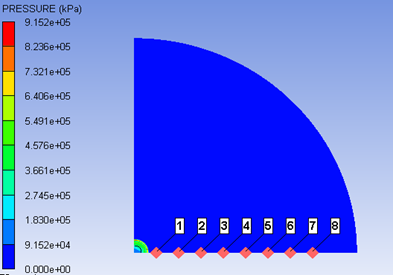Figure 1. AUTODYN model

Explosives TNT and RDX were sphere charged, positioned at the center of the coordinates. The initiation points were all at the center of the coordinates. The water range of the calculating model was 1m for the radius. X axis was symmetry axis. The explosive doses were 10 g, 30 g, 50 g and 70 g, respectively. Based on the volume of the 3-dimensional model, the charge radius size of the 3-dimensional model was applied to the simulation of 2-dimensional model. According to the charge density 1.63 g/cm3 of TNT, 1.185 g/cm3 of RDX, the radiuses of charge is calculated below, as shown in Table 1.

Table 1. Different radius corresponding different doses

 TNT charge quantity (g) Charge radius (mm) RDX charge quantity (g) Charge radius (mm) 10 9.2 10 10.15 30 13.3 30 14.5 50 15.75 50 17.4 70 17.5 70 19.4

2.2 Material model and equations of states

Two substances (water and explosive) were considered during the simulation of underwater explosion. There are two types of state equations. Shock equation chosen as the state equation of water and JWL equation chosen as the state equation of explosive.

2.2.1 The state equation of water

Shock state equation was employed in the external environment of the water when calculating the model.

When the sludge-water environment compressed (μ>0), the state equation was as following:

$P={{A}_{1}}\mu +{{A}_{2}}{{\mu }^{2}}+{{A}_{3}}{{\mu }^{3}}+({{B}_{0}}+{{B}_{1}}\mu ){{\rho }_{0}}e$     (1)

When the sludge-water environment expanded (μ<0), the state equation was:

$P={{T}_{1}}\mu +{{T}_{2}}{{\mu }^{2}}+{{B}_{0}}{{\rho }_{0}}e$     (2)

When the sludge-water environment unchanged (μ=0), the state equation was:

$P={{B}_{0}}{{\rho }_{0}}e$     (3)

In these equations, P stands for water pressure, μ stands for compression ratio, μ=ρ/ρ0-1; e stands for internal energy of water; ρ0 stands for water density, ρ0=1g/cm3. A1=2.2×106 kPa, A2=9.54×106 kPa, A3=1.457×107 kPa, B0=B1=0.28, T1=2.2×106 kPa, T2=0.

The shock state equation of water was as following:

$P={{P}_{H}}+\frac{\Gamma }{\nu }(e-{{e}_{H}})$     (4)

The relationships between underwater explosion shockwave and each parameter were as follow:

${{P}_{H}}=\frac{{{\rho }_{0}}{{\delta }^{2}}\mu (1+\mu )}{{{[1-(\lambda -1\mu )]}^{2}}}$     (5)

${{e}_{H}}=\frac{1}{2}\frac{{{P}_{H}}}{{{\rho }_{0}}}\frac{\mu }{(1+\mu )}$     (6)

In these formulas, λ and δ are constants. The relationship of them was determined by the Eq(7):

$D=\lambda u+\delta$     (7)

In Eq(7), D is the shockwave velocity, and u is the particle velocity after shockwave. ρ0=0.998 g/cm3, C0=1.647×103 m/s, λ=1.921

2.2.2 The state equation of explosive

The standard JWL state equation was chosen for the explosive, and the specific form of the state equation is as follows:

$P=A\left( 1-\frac{\omega }{{{R}_{1}}\bar{V}} \right){{e}^{-{{R}_{1}}\bar{V}}}+B\left( 1-\frac{\omega }{{{R}_{1}}\bar{V}} \right){{e}^{-{{R}_{2}}\bar{V}}}+\frac{\omega E}{{\bar{V}}}$     (8)

In Eq(8), P stands for the shockwave pressure,V stands for the relative specific volume, E is the internal energy per unit volume on the initial. For the TNT explosive, the coefficients of the equation of state are: A=3.7377 Mbar, B=0.03741 Mbar, R1=4.15, R2=0.9, ω=0.35, V =1.0.

2.3 AUTODYN simulation

AUTODYN software was employed for the simulation of underwater explosion. For the types of explosives, TNT and RX-01 were chosen. In a sphere water area with a radius of 1m, the influence of the numerical simulation of underwater explosion was carried out under various conditions. And then the data results and conclusions analysis were obtained.

To study the effect of different types of explosives (TNT and RDX) on underwater explosion, as well as the impact of different charges (10 g, 30 g, 50 g and 70 g), in the two cases,8 cases of comparative simulations were conducted;

To study the influence of different meshing densities on the physical properties of real explosives during underwater explosion, three meshing sizes: 2 mm, 2.5 mm and 4 mm were investigated.

To study the effect of different initiation points on underwater explosion, two initiation types (center point initiated and upper extreme point initiated) were set up in the same model, and the peak values of shockwave of the two types were compared;

To study the influence of different model sizes on underwater explosion, the explosion simulation of  different charge (10 g, 30 g, 70 g, 50 g) explosives (RDX and TNT), namely 8 groups, were carried out in a sphere water area with a radius of 0.5 m.

3. Results and Discussion

3.1 Explosives types and explosive doses

Considering different explosive doses (10 g, 30 g, 50 g and 70 g) and different explosive types (TNT, RDX), the shockwave propagation process of underwater explosion was simulated in a quarter circular closed water area with a radius of 1 m. In this study, the explosion of explosives was in an infinite water medium, so there was a certain gap between the calculating values and the theoretical values. In that case, we need to consider the influence of two factors, namely the peak pressure and the impulse of the shockwave, impacting on the cracking of sludge. The case of explosion of 50 g column shaped TNT in a quarter circular water area with a radius of 1 m was chosen for instance. Boundary condition was Transmitting Boundary. The model was divided into 500 grids for both X, Y directions.

The empirical formulas of underwater explosion field were established based on the fitting of the similar rate of explosion and the experimental data of underwater explosion. As shown in Figure 2, before 0.3 m, the difference between pressure and impulse of the calculating value and the empirical value was relatively big, after 0.3 m, the difference was smaller. Because of the influence of Euler meshing, the empirical values of the impulse of shockwave were larger than the calculating values, but the calculating values of pressure value were closer to the empirical values. Therefore, the simulation data of this study was effective.

Simulation and comparative analysis of different explosive doses were conducted using TNT in a quarter circular water area with a radius of 1 m ,the center of the explosive and the origin of the coordinates of the model were overlap, the initiation point was also set there. The full waveform of 30 g TNT explosive was chosen as an example, the waveforms of 10 g, 50 g and 70 g TNT simulation of the process are similar. So just cite this one full waveform.

## 2.pngFigure 2. Pressure and impulse distribution of shockwave

## 3.pngFigure 3. Pressure-time waveform of TNT shockwave

As is shown in Figure 3, the peak value of the shockwave decreased with the increase of the distance from the explosion center, the measurement point at 0.1 m was nearest to the center of explosion, therefore the front section of the shockwave did not receive much interference from water environment, the waveform changed normally. However, curve after positive pressure due to the influence of the water resistance, the shockwave would show a decreasing trend. Between detector 1 and detector 8, the shockwave attenuation was very fast, in the time of 0.5 ms, the energy level decayed for 105 orders of magnitude. And through the result from the detector at 0.8 m, after the shockwave attenuated to the initial pressure, shockwave pressure will be relatively stable and very slow down.

By the data from each detection point in Figure 4, it could be found that although the rates of attenuation were different, the final shockwave peak pressure would decay to the initial pressure value of water under each explosive dose of TNT. And it could be seen that between 0.1 m and 0.2 m, energy release of shockwave is the most obvious.

## 4.pngFigure 4. Shock wave pressure values at different locations of TNT

3.2 Meshing

Selecting 10 g TNT explosive, in a two dimensional closed 1/4 sphere water area with a radius of 1 m, numerical simulation was employed to study the influence of grid size on explosion shockwave value. Grid sizes were selected as 2 mm, 2.5 mm and 4 mm. The simulation also used the grid of 1 mm. However, due to the large error accumulation of energy calculation, the simulation calculation could not be carried out smoothly. So, the 1 mm grid size was too small for this research model. Taking the introduction of artificial viscosity into account, the grid meshing should not be too large. And in order to achieve a better accuracy to ensure the pressure precision information of the shockwave front, the meshing must be small enough. The resistance of air to the shockwave was much lower than that of water, the peak value of shockwave attenuated according to the exponential law.

The simulation data of 3 sizes of grids are shown in Table 2. As is shown in Table 2, the smaller the grid, the more accurate the numerical simulation of the shockwave, and in the same distance, the larger the peak value of shockwave would be. The error of 2 mm meshing was more stable than that of the other two.

3.3 Initiating position

In this section, the modeling of AUTODYN-2D two-dimensional X, Y axis symmetry, 1/4 sphere model water area with a radius of 1m, 70 g TNT spherical charge was employed, TNT ball radius was 17.5 mm, placed at the origin of coordinates, the TNT sphere center and the origin of coordinates is coincide. Boundary condition was Transmitting Boundary. To record the change of shockwave pressure value over time, 8 points were set on the X axis at intervals of 0.1 m from 0.1 m to 0.8 m, interval of 0.1 μs. The model of explosive placement is shown in Figure 5(a), red dot is the initiating point, one of the first initiation at the sphere center, the second in the Y axis of the upper boundary point.

Figure 5(b) gives the data comparison chart of the shockwave pressure generated by the explosion at the center point of the explosive and the end point of the Y axis corresponds to the position. . At the beginning within 0.4 m, the pressure difference between the two was relatively large, the initiation at the upper end point of Y axis achieved higher pressure than center point, while in the second half, the gap between the peak pressures of the two gradually reduced, and finally almost close.

Table 2. Error between empirical value and simulative value

 Distances (m) Empirical value of shockwave (MPa) simulation value of shockwave (MPa) 2mm error% 2.5mm error% 4mm error% 0.1 109 114 4.6 111 1.8 91.5 -16.0 0.2 42.76 44.71 4.5 43.72 2.25 37.08 -13.3 0.3 25.59 26.23 2.5 25.72 0.5 22.01 -14.0 0.4 17.91 18.12 1.2 17.89 -0.1 15.18 -15.2 0.5 15.01 13.64 -9.1 13.29 -11.5 11.46 -23.7 0.6 12.13 10.88 -10.3 10.54 -13.1 9.12 -24.7 0.7 10.24 8.88 -13.3 8.66 -15.4 7.46 -27.1 0.8 8.93 7.53 -15.7 7.30 -18.3 6.36 -28.8

## 5.png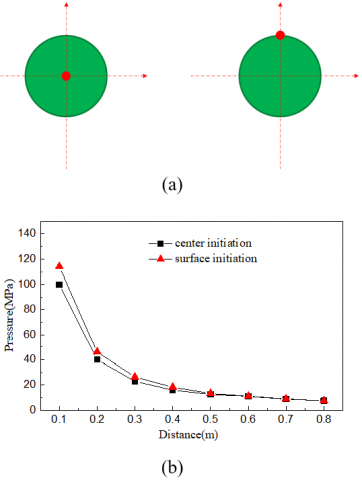Figure 5. Schematic diagram of initiating position and pressure of different initiation points

3.4 Container size

Considering the effect of the superposition of the reflected wave and the incident wave, how will the peak of the shockwave change if the explosive path being shortened? Similarly, TNT and RDX were selected with the explosive doses being 10 g, 30 g, 50 g and 70 g, respectively. The simulation was carried out in a 1/4 sphere model with a radius of 0.5 m. Explosives were placed at the origin of the coordinates, so was the initiation position. Boundary condition was chosen as Transmitting Boundary. As shown in Figure 6, the detectors were set in the water to detect the pressure value of the shockwave,5 detecting points were set at 0.1 m to 0.5 m from the initiating point evenly, the detector at 0.5 m was to record the impact of reflection wave on the shockwave. The detectors recorded data once every 1 μs.

## 6.png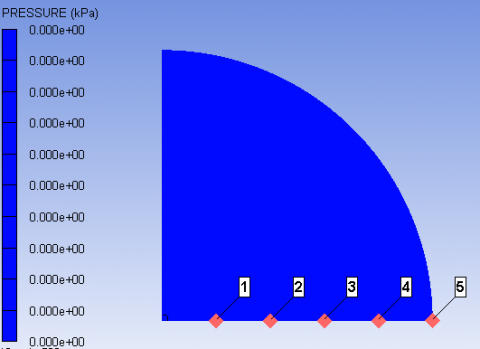Figure 6. Distribution of detectors in 0.5m sphere water area

## 7.png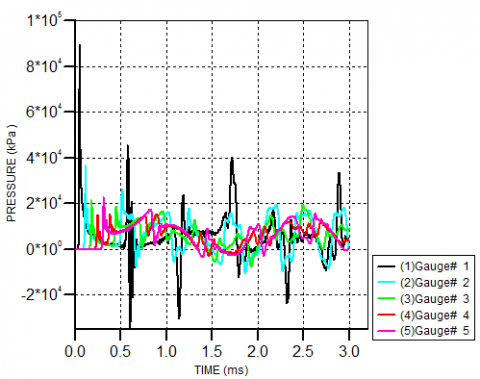Figure 7. Pressure-time of RDX shockwave in 0.5m sphere water area

In this section, the simulation result of RDX is selected for illustration. As shown in Figure 7, the detecting point at 0.1 m was nearest to the center of explosion, therefore the front section of the pressure shockwave did not receive much interference from water environment, the waveform changed normally. However, curve after positive pressure due to the influence of the water resistance, the shockwave would show a decreasing trend. Between detector 1 and detector 4, the shockwave attenuation was very fast, but the shockwave attenuation was not as much as that in the 1 m water area. It means that, before decay to a higher value, reflected wave had touched the wall after reflection rebound and superposed with the incident wave. The detector at 0.5 m showed that, peak value would increase after the incident wave superposed with the reflected wave. And because of the explosion shockwave path was as compared to 1 m shorter than twice as much, at the same duration (3 ms), the frequency and degree of negative pressure increased obviously. Also found from the detector at 0.5 m, the larger the explosive dose, the faster the shockwave propagated, the earlier the wave front would touch the container wall. This rule is the same as that of TNT, so in the same model, the law of the peak attenuation rate of TNT and RDX is certain.

## 8.png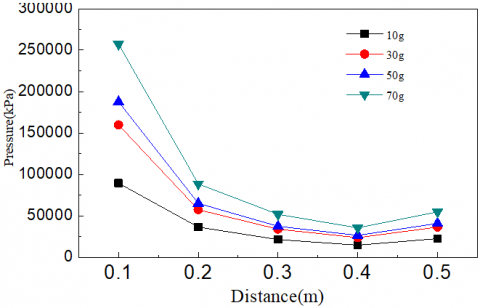Figure 8. Shockwave pressure values at different locations of RDX

As shown in Figure 8, under each explosive dose, the attenuation degree of peak value of shockwave pressure decreased with the distance from the explosion center getting longer. Compared with TNT, pressure values were not significantly different. Between 0.1 m and 0.2 m, the pressure value of the explosion shockwave attenuated the most, and the maximum energy was released.

As shown in Figure 9, under each explosive dose of TNT, comparison of peak pressure between 0.5 m and 1 m container model was chosen as example. According to the comparison of the peak value of shockwave in different water area, we could know whether the size of the container had a certain effect on the shockwave, we know that whether it was TNT or RDX, in the model of 0.5 m water area, the pressure peak value of the shockwave was smaller than that of the value in the water area of 1 m. Therefore, the change of container size will affect the peak pressure of shockwave, but the extent of the impact will not be great.

## 9.png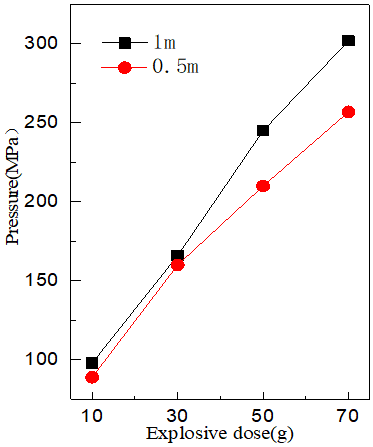Figure 9. Shockwave pressure value in 1 m and 0.5 m containers

4. Conclusions

Shockwave will quickly decay to the initial static pressure within a relatively short time (0.5 ms) and a short distance (0.2 m) in the water area. The larger the explosive dose, the greater the attenuation of the shockwave will be.

When the simulation grid meshed into relatively small sizes, the simulation values of the shockwave will be relatively higher than the empirical values at the same distance, and the accuracy will also be higher, but the computation will increase exponentially; Compared with 2.5 mm and 4 mm, the error of 2 mm meshing was relatively smaller, the calculation quantity and precision were better; And, the 1 mm meshing had the case of excessive accumulation of error energy, so 2 mm was the best.

Comparing the upper end point of Y axis initiation with the central initiation, the initial pressure of the shockwave will be different. The shockwave pressure value of the central initiation was smaller than that of the upper end point of Y axis initiation.

The initial shockwave peak value of the radius of 0.5 m sized model was about 10 MPa smaller than that of the 1 m sized model, but with respect to the explosive energy level of TNT and RDX, the influence can be ignored. The shockwave attenuation degrees of the two models were the same. Within the same time, incident wave and reflected wave superposition of the 0.5 m container model is more than that of the1 m model.

Acknowledgment

This study was jointly supported by the Research Fund for the Doctoral Program of Higher Education of China (20130042110009), the Fundamental Research Funds for the Central Universities of China (N140306001) and National Natural Science Foundation of China (51178098).

References

 P. Cole, Underwater Explosion, New Jersey, USA: Princeton University Press, 1948.

 M. Michael, “Explosion effects and properties: Part II. Explosion effects in the water,” National Surface Warfare Center Report, AD-A 056694, 1978.

 P. Smith and J. Hetherington, “Blast and ballistic loading of structures,” Butterworth-Heinemann Ltd, 1994.

 S. K. Chan, “An improvement in the modified finite element procedure for underwater shock analysis,” in Proc. of the 61st Shock and Vibration Symposium, San Diego, CA, 3, 1990, pp. 87-101.

 S. Fu, Z. P. Wang, Z. S. Zhang, G. X. Cui and R. S. Li, “Numerical study on underwater explosion of near surface,” (in Chinese), Chinese Journal of Mechanics, vol. 27, no. 3, pp. 267-276, 1995.

 H. Z. Zhao, “The effect of water in the open space on the blasting shockwave,” (in Chinese), Explosion and Shock Waves, vol. 21, no. 1, pp. 26-28, 2001. DOI: 10.3321/j.issn:1001-1145.2001.01.006.

 B. L. Sun, W. B. Gu, J. P. Jiang and T. H. Yang, “Numerical simulation research of two explosion charges at the bottom in shallow water,” (in Chinese), Explosion and Shock, vol. 23, no. 5, pp. 460-465, 2003. DOI: 10.3321/j.issn:1001-1455.2003. 05. 013.

 N. V. Petrov and A. A. Schmidt, “Mathematical modeling of underwater explosion near free surface,” Technical Physics Letters, vol. 37, no. 5, pp.445-448, 2011. DOI: 10.1134/S1063785011050270.

 W. Cao, Z. He and W. Chen, “Experimental study and numerical simulation of the afterburning of TNT by underwater explosion method,” Shock Waves, vol. 24, pp. 619-624, 2014. DOI: 10.1007/s00193-014-0527-2.

 N. V. Petrov and A. A. Schmidt, “Multiphase phenomena in underwater explosion,” Experimental Thermal and Fluid Science, vol. 60, pp. 367-373, 2015.

 A. Daramizadeh and M. R. Ansari, “Numerical simulation of underwater explosion near air-water free surface using a five-equation reduced model,” Ocean Engineering, vol. 110, pp. 25-35, 2015.